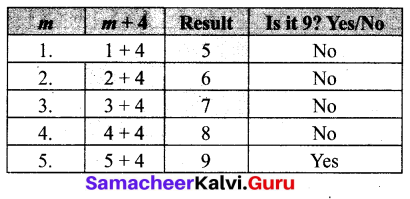## Tamilnadu Samacheer Kalvi 6th Maths Solutions Term 1 Chapter 2 Introduction to Algebra Intext QuestionsTry These (Text hook Page No. 41)

Question 1.
Observe the following patterns and complete them.
(i) 5, 8, 11, 14, ___, ____, ____
(ii) If 15873 × 7 = 111111 and 15873 × 14 = 222222 then, what is 15873 × 21 = ? and 15873 × 28 = ?
Solution:
(i) 17, 20, 23
Hint: 5 + 3 = 8, 8 + 3 = 11, 11+3 = 14
(ii) 15873 × 21 = 333333; 15873 × 28 = 444444
Hint: 15873 × 14 = 15873 × 7 × 2 = 111111 × 2 = 222222

Question 2.
Draw the next two patterns and complete the table.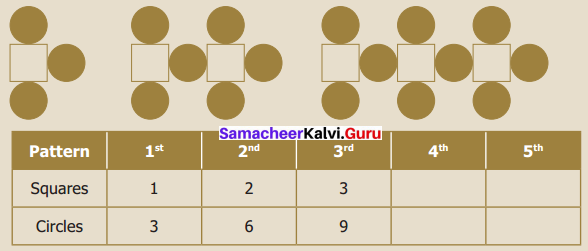Solution:
The next two patterns: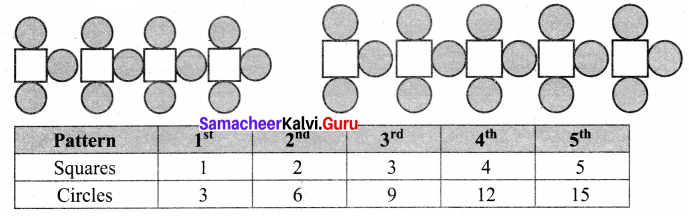Question 3.
Create your own patterns of shapes and prepare a table.
Solution:
(i) Match stick pattern of triangles.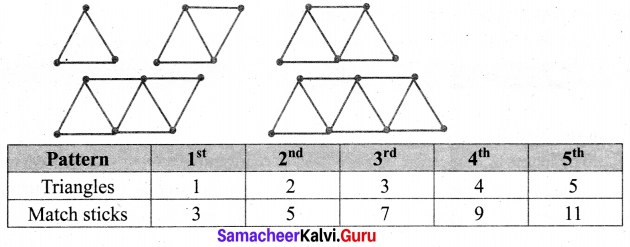(ii) Pattern of squares and circles.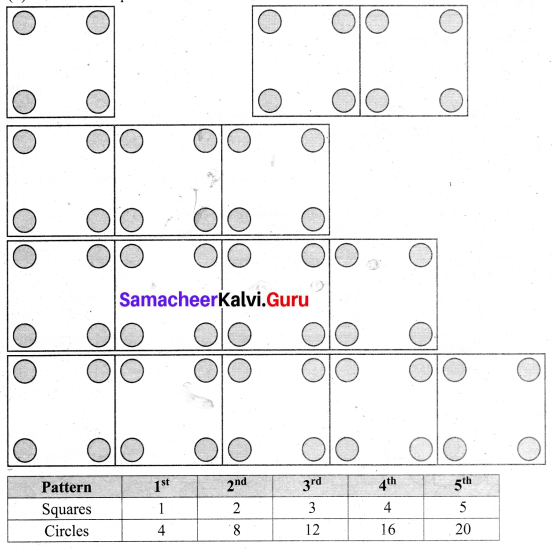Try These (Text hook Page No. 46 to 48)

Question 4.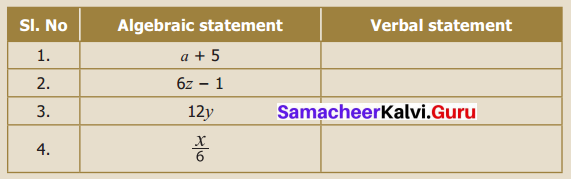Solution: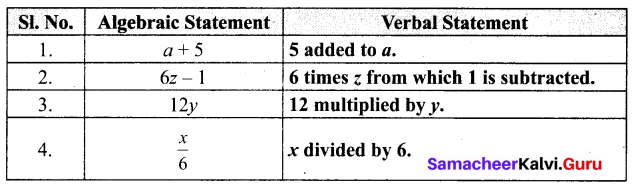Question 5.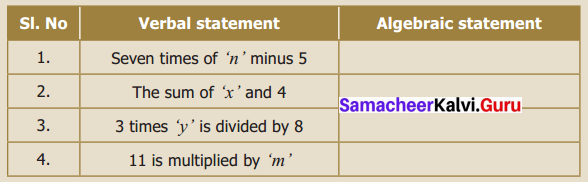Solution: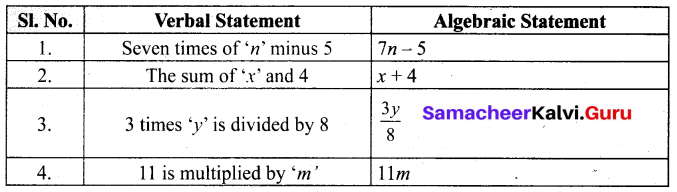Question 6.
Find the unknown.
(i) 37 + 43 = 43 + ____
(ii) (22 + 10) + 15 = ____ + (10 + 15)
(iii) If 7 × 46 = 322, then 46 × 7 = _____
Solution:
(i) 37 + 43 = 43 + 37
(ii) (22 + 10) + 15 = 22 + (10 + 15)
(iii) If 7 × 46 = 322, then 46 × 7 = 322

Question 7.
Find the suitable value of ‘m’, to get a sum of 9?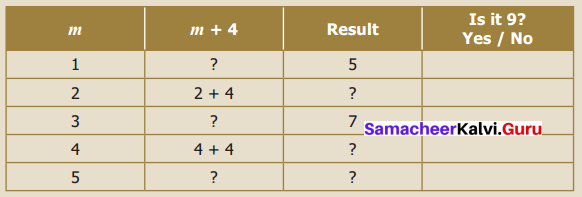Solution: